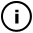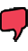# Saturday, January 23, 2010

## Spirabone with halo

// modified from a sketch from Orgicus

float c = 0;
float r = 0;
float s = 1, x,y;
int numberOfFrames = 0;

void setup(){
background(0xFFFFFF);
size(800,600);
smooth();
colorMode(HSB);
noStroke();

}

void draw(){
numberOfFrames++;

if(numberOfFrames > 5500) return;

translate(width * .5, height * .5);

if(x < 500 && y < 500){
s = 1 + random(15);
stroke(255,125,125,90);
//stroke((sin(numberOfFrames/50)+1)*255/2,255,255);
//line(x,y,random(-5,5),random(-5,5));

line(x,y,2,2);

//c += 1 - r/500;
c += 1 - log(r+10)/10;
r = (4500-numberOfFrames)/10.0 + sin((4500-numberOfFrames)/6.0)*10.0;
}
}

## infosubmitted by: DARYL_Gamma
views:This sketch has a parent
Tags: spiral, rainbow

## commentsloading...